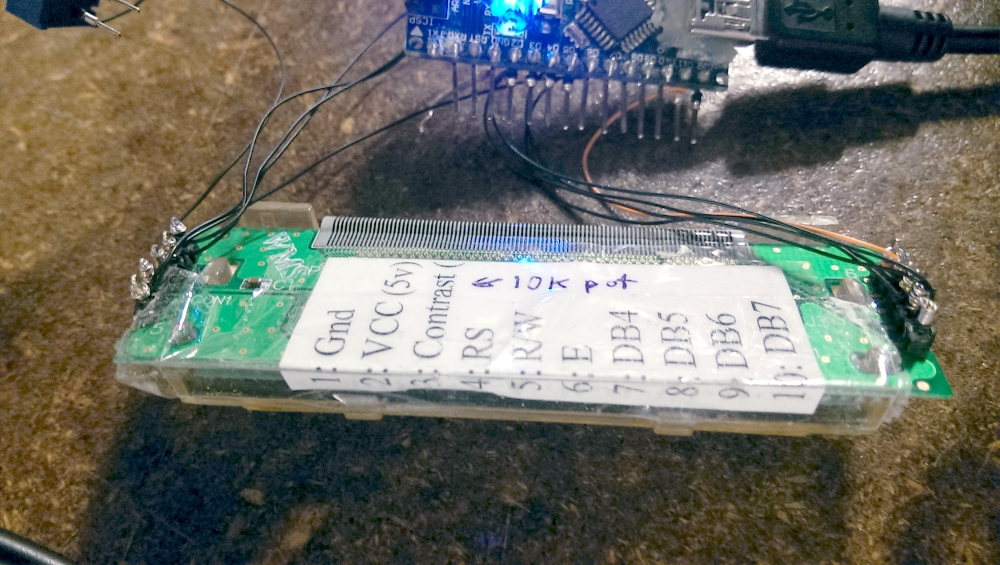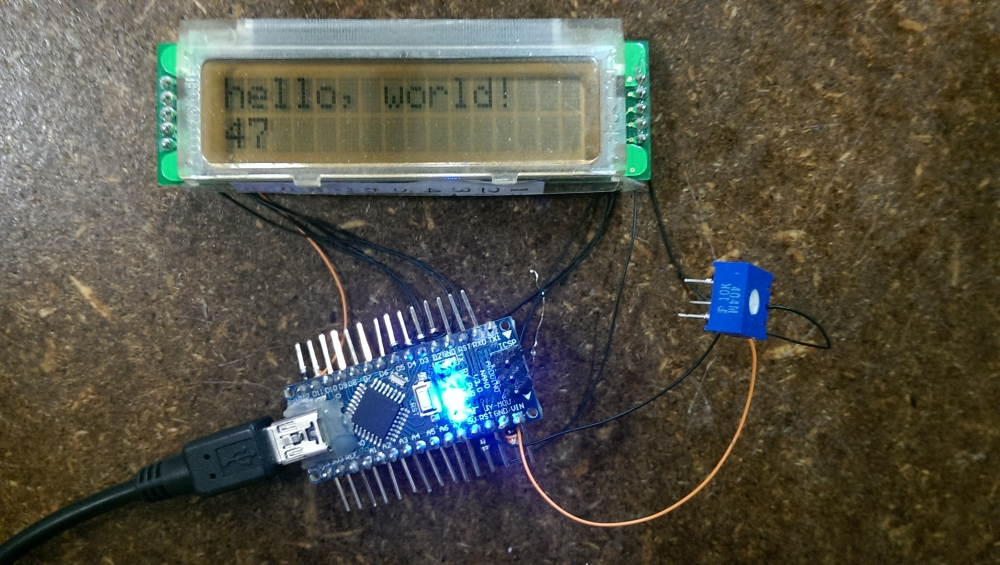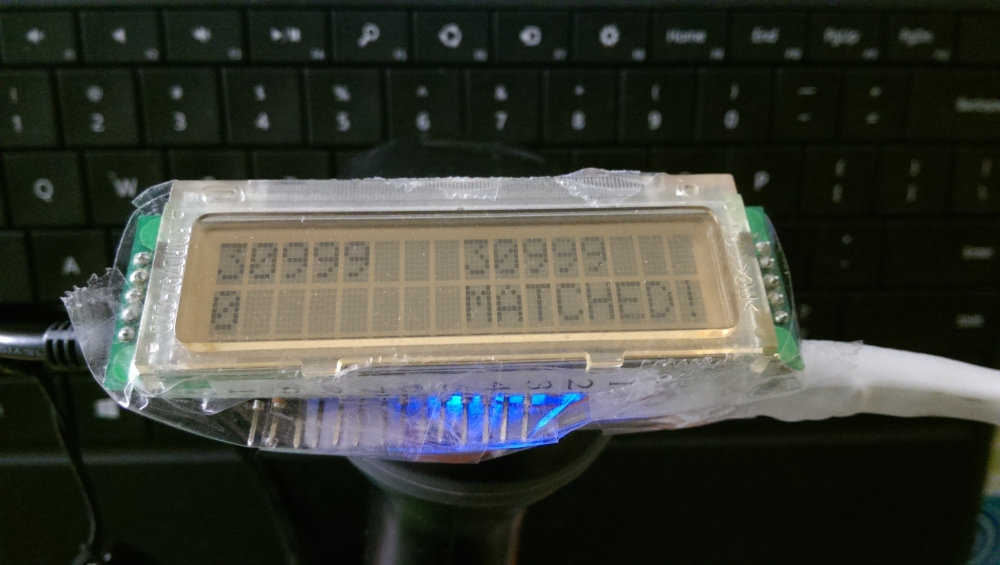This a continuation of working out how to interface with the MJ-4209 linear barcode reader. Quick writeup located in this post

In this project, my objective is to find an item from a set of sequentially barcoded array of shelved item. Let’s call it a random access bookshelf. Where every book is assigned a number, and arranged in that order. Each book has the number encoded as a barcode stuck to the book as well.

In this example, we have a print out of items we need, along with it’s barcode. We cannot interface directly with the database. So instead, what we want, is to assign a distance of items between your current book, and the book you want.

To assist in this, I used a salvaged 16x2 LCD screen, wired to an arduino nano, and hooked to the barcode reader via serial.

This is by first determining the pinout of the LCD screen, by observing the layout of the PCB traces (And using the silkscreen text as hints. E.g. which one is the contrast pin? “CON1” sounds like it.). Once that is done, its time to hook up the screen, and testing that it works with an hello world sketch.Next is to hook up the barcode reader to the serial input of the arduino nano, and start coding up the interface.The display is assigned like this (Scanning a barcode twice, sets that barcode as “Reference Barcode”) :

< Reference Barcode >		< Current Barcode Scanned >
< Distance to reference>	< Visual indicator of distance>Where the “Distance to reference” is essentially “Reference Barcode”-“Current Barcode”. This gives the user a rough estimate of where to walk to reach the desired book. The visual indicator essentially is a visual version of the “Distance to reference” field, showing icons instead of numbers, so that humans can parse what to do faster (we see icons faster than numbers). E.g. “«” may mean to move left along the bookshelf, while “»” may mean to walk right along the bookshelf. This is easer to read than “24” or “-32” as an indicator of where to walk.

Attached is a copy of the code used to run the arduino nano. There is a setup, where we set up the LCD and serial interface (for barcode reader). A main loop, which checks for any new inputs. If there is, then it processes, and then displays the new results.

TIPS: In general, it is not recommended to place the refresh display in the main loop. This is because clearing and redrawing the screen takes time, and doing this too often will make the screen look too faint. Also it is likely to use up more power this way, since you are actively refreshing the LCD screen too often.

# results found

### int overflow

• Noticed buffer overflow of integers. Realized that int is restricted to (-32,768 to 32,767 ), and the barcode in field may be larger than that 342374. Solution is to increase memory size of int to “long int” ( range from -2,147,483,648 to 2,147,483,647).

### arrow indicator pointing wrong way

• Noticed that the arrow is pointing the wrong way, so reversed the direction. This is since the system is ment to look like this:

  --------------------------------> books serial incremented from left to right

(1) (2) (3) (4) (5) (6) (etc...)
^		^			^
">"		"ref"		"<"


This will be reversed if books are arranged from left to right

### indicator > is sort of hard to understand.

So modified to provide this kind of interface for indicating general direction to walk.

}--||---
>--||---
->-||---
-->||---
--->|---
>>>!!<<<
---|<---
---||<--
---||-<-
---||--<
---||--{


Which is easier to read than V1’s

>>>|
>>|
>|
|<
|<<
|<<<


### Can’t be lazy coding for the » « comparison display

Instead of lazy coding like this:

if( distance == 0 ){
lcd.print("MATCHED!");
} else if( distance > 100000 ){
lcd.print(">--||---");
} else if ( distance >  10000 ){
lcd.print("->-||---");
} else if ( distance >  1000 ){
...


Which sort of does work, but is frankly not accurate near the middle. You should code it like

if( distance == 0 ){
lcd.print(">>>!!<<<");
} else if( distance>10000 ){
lcd.print("}--||---");
} else if (  10000>=distance   &&   distance>1000      ){
lcd.print(">--||---");
} else if (   1000>=distance   &&   distance>100       ){
...


Takes a bit more work, but is easier to read the logic behind it.

### Want to indicate if in “new ref barcode, so please scan your first barcode”

To tackle this, you want multiple “views” or screens that you can switch to based on modes. So this was implemented by using enumerated type, and switch cases to deal with switching between, hello screen, new reference barcode detected, normal scanning loop.

### Want to indicate clearly if matched, in distance field, now that you changed the » « system.

Just need a simple check in if( distance == 0 ){ to activate a LCD writing command that would replace the distance field with “MATCHED” message.

if( distance == 0 ){
lcd.print(">>>!!<<<");
lcd.setCursor(0, 1);
lcd.print("MATCHED ");
}


## Coding V2

So just took these fixes into V2 and it works fine now

/*

Sequential barcode finder
Author: Brian Khuu

Based on the liquid crystal barcode examples.
Compare current barcode to a reference barcode. Good for finding items barcoded in a numerically sequential manner.

V2 - forgot that int in arduino is restricted to (-32,768 to 32,767 ), so integer overflow occur when storing number 342374.
Also, <<< and >>> needs to be reversed, since the ware house counts upwards in the right side direction.

*/

// vars

long int referencebarcode=0;
long int currentbarcode=0;
long int previousbarcode=0;
long int distance=0;

enum mode_type {
FIRST_RUN,
NEW_REF, // Used when we are just waiting for our first barcode.
SEARCH
};

enum mode_type mode=FIRST_RUN;

// include the library code:
#include <LiquidCrystal.h>

// initialize the library with the numbers of the interface pins
LiquidCrystal lcd(12, 11, 5, 4, 3, 2);

void setup(){
// set up the LCD's number of columns and rows:
lcd.begin(16, 2);
// initialize the serial communications:
Serial.begin(9600);
// Welcome and help message
lcd.print("Scan Twice To");
lcd.setCursor(0, 1);
lcd.print("Set Ref Barcode");
}

void loop()
{
if (Serial.available()) {

/*
PROCESS INPUT
*/
delay(100); // wait a bit to fill buffer.
//Read in current barcode (and save previous barcode)
/*
CHECK IF READ CORRECTLY, IF SO. THEN PROCESS INPUT
*/
// Had issue with double trigger, so maybe an end of line detect is needed. (perhaps parse int saw "<num>\r\n" as two integers ending with "\n" and "\r" respectively)
// save detected value, otherwise discard it.
previousbarcode = currentbarcode;
//Check if same barcode is read twice ( In this context, it means set to new reference barcode )
if( previousbarcode == currentbarcode ){
referencebarcode = currentbarcode;
currentbarcode = 0;
// switch mode to new reference barcode, to prompt user to scan first barcode
mode =  NEW_REF;
} else {
mode =  SEARCH;
}
}

/*
DISPLAY
note: you don't want this in main loop, otherwise you refresh it too fast, and the contrast of the display will go down as a reault (plus it sounds like you might eat up more power too)
*/
switch(mode){

case  NEW_REF :
lcd.clear();
// New ref barcode mode! Show instruction!
lcd.setCursor(0, 0);
lcd.print("New Ref Barcode:");
lcd.setCursor(0, 1);
lcd.print(referencebarcode);
lcd.setCursor(8, 1);
lcd.print("SCAN NOW");
break;

case  SEARCH :
lcd.clear();
// Current barcode we are looking for
lcd.setCursor(0, 0);
lcd.print(referencebarcode);
// Show barcode we scanned
lcd.setCursor(8, 0);
lcd.print(currentbarcode);
// Distance from that barcode
lcd.setCursor(0, 1);
distance = referencebarcode-currentbarcode;
lcd.print(distance);
// Show distance to barcode we want
lcd.setCursor(8, 1);
if( distance == 0 ){
lcd.print(">>>!!<<<");
lcd.setCursor(0, 1);
lcd.print("MATCHED ");
} else if( distance>10000 ){
lcd.print("}--||---");
} else if (  10000>=distance   &&   distance>1000      ){
lcd.print(">--||---");
} else if (   1000>=distance   &&   distance>100       ){
lcd.print("->-||---");
} else if (    100>=distance   &&   distance>10        ){
lcd.print("-->||---");
} else if (     10>=distance   &&   distance>8         ){
lcd.print("--->_---");
} else if (      8>=distance   &&   distance>6         ){
lcd.print("-->>_---");
} else if (      6>=distance   &&   distance>4         ){
lcd.print("->>>_---");
} else if (      4>=distance   &&   distance>0         ){
lcd.print(">>>>_---");
} else if (      0>=distance   &&   distance>-4       ){
lcd.print("---_<<<<");
} else if (     -4>=distance   &&   distance>-6       ){
lcd.print("---_<<<-");
} else if (     -6>=distance   &&   distance>-8       ){
lcd.print("---_<<--");
} else if (     -8>=distance   &&   distance>-10       ){
lcd.print("---_<---");
} else if (    -10>=distance   &&   distance>-100      ){
lcd.print("---||<--");
} else if (   -100>=distance   &&   distance>-1000     ){
lcd.print("---||-<-");
} else if (  -1000>=distance   &&   distance>-10000    ){
lcd.print("---||--<");
} else if ( -10000>=distance ){
lcd.print("---||--{");
}

break;
}

/*DEBUG*/
Serial.print(referencebarcode);    Serial.print(",");    Serial.print(previousbarcode);    Serial.print(",");    Serial.print(currentbarcode);     Serial.print(",");    Serial.print(distance);    Serial.print("\n");
}

}



Let me know if you found this useful!